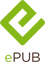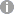# Derivative with a New Parameter

Theory, Methods and Applications

• 1. Auflage
• |
• erschienen am 18. September 2015
• |
• 170 Seiten

 E-Book | ePUB mit Adobe DRM | Systemvoraussetzungen
978-0-12-803825-3 (ISBN)

Derivative with a New Parameter: Theory, Methods and Applications discusses the first application of the local derivative that was done by Newton for general physics, and later for other areas of the sciences.

The book starts off by giving a history of derivatives, from Newton to Caputo. It then goes on to introduce the new parameters for the local derivative, including its definition and properties. Additional topics define beta-Laplace transforms, beta-Sumudu transforms, and beta-Fourier transforms, including their properties, and then go on to describe the method for partial differential with the beta derivatives.

Subsequent sections give examples on how local derivatives with a new parameter can be used to model different applications, such as groundwater flow and different diseases. The book gives an introduction to the newly-established local derivative with new parameters, along with their integral transforms and applications, also including great examples on how it can be used in epidemiology and groundwater studies.

• Introduce the new parameters for the local derivative, including its definition and properties
• Provides examples on how local derivatives with a new parameter can be used to model different applications, such as groundwater flow and different diseases
• Includes definitions of beta-Laplace transforms, beta-Sumudu transforms, and beta-Fourier transforms, their properties, and methods for partial differential using beta derivatives
• Explains how the new parameter can be used in multiple methods
 Reihe: Sprache: Englisch Verlagsort: London | Großbritannien Verlagsgruppe: Elsevier Science Dateigröße: 5,36 MB Schlagworte: ISBN-13: 978-0-12-803825-3 (9780128038253) ISBN-10: 0-12-803825-X (012803825X)weitere Ausgaben werden ermittelt
• Intro
• Title page
• Dedication
• Preface
• Acknowledgments
• Chapter 1: History of derivatives from Newton to Caputo
• Abstract
• 1.1 Introduction
• 1.2 Definition of local and fractional derivative
• 1.3 Definitions and properties of their anti-derivatives
• 1.4 Limitations and strength of local and fractional derivatives
• 1.5 Classification of fractional derivatives
• Chapter 2: Local derivative with new parameter
• Abstract
• 2.1 Motivation
• 2.2 Definition and anti-derivative
• 2.3 Properties of local derivative with new parameter
• 2.4 Definition of partial derivative with new parameter
• 2.5 Properties of partial beta-derivatives
• Chapter 3: Novel integrals transform
• Abstract
• 3.1 Definition of some integral transform operators
• 3.2 Definition and properties of the beta-Laplace transform
• 3.3 Definition and properties of the beta-Sumudu transform
• 3.4 Definition and properties of beta-Fourier transform
• Chapter 4: Method for partial differential equations with beta-derivative
• Abstract
• 4.1 Introduction
• 4.2 Homotopy decomposition method
• 4.3 Variational iteration method
• 4.4 Sumudu decomposition method
• 4.5 Laplace decomposition method
• 4.6 Extension of match asymptotic method to fractional boundary layers problems
• 4.7 Numerical method
• 4.8 Generalized stationarity with a new parameter
• Chapter 5: Applications of local derivative with new parameter
• Abstract
• 5.1 Introduction
• 5.2 Model of groundwater flow within the confined aquifer
• 5.3 Steady-state solutions of the flow in a confined and unconfined aquifer
• 5.4 Model of groundwater flow equation within a leaky aquifer
• 5.5 Model of Lassa fever or Lassa hemorrhagic fever
• 5.6 Model of Ebola hemorrhagic fever
• Bibliography
Chapter 1

# History of derivatives from Newton to Caputo

## Abstract

In this chapter, we have presented the history of fractional derivative from Newton to Caputo. We show different definitions of local and fractional derivatives. We recall some important theorems of these derivatives. We present the properties of their associated anti-derivatives. We also present the limitations, advantages, and disadvantages of each derivative. We propose a list of criteria that need to be satisfied by an operator to be classified as a derivative. Using these criteria, we have classified all operators that are claimed to be derivative in the literature.

Keywords

Local and Fractional derivatives

Criteria of a fractional derivative

## 1.1 Introduction

In mathematics, differential calculus is a subfield of calculus concerned with the study of the rates at which quantities change. It is one of the two traditional divisions of calculus, the other being integral calculus. The primary objects of study in differential calculus are the derivative of a function, related notions such as the differential, and their applications. The derivative of a function at a chosen input value describes the rate of change of the function near that input value . The process of finding a derivative is called differentiation. Geometrically, the derivative at a point is the slope of the tangent line to the graph of the function at that point, provided that the derivative exists and is defined at that point. For a real-valued function of a single real variable, the derivative of a function at a point generally determines the best linear approximation to the function at that point. The concept of a derivative in the sense of a tangent line is a very old one, familiar to Greek geometers such as Euclid (c. 300 BC), Archimedes (c. 287-212BC) , and Apollonius of Perga (c. 262-190BC) [3-5]. Archimedes also introduced the use of infinitesimals, although these were primarily used to study areas and volumes rather than derivatives and tangents; see Archimedes' use of infinitesimals.

The use of infinitesimals to study rates of change can be found in Indian mathematics, perhaps as early as AD500, when the astronomer and mathematician Aryabhata (476-550) used infinitesimals to study the motion of the moon. The use of infinitesimals to compute rates of change was developed significantly by Bhskara II (1114-1185); indeed, it has been argued that many of the key notions of differential calculus can be found in his work, such as "Rolle's theorem." The Persian mathematician, Sharaf al-Dn al-Ts (1135-1213) , was the first to discover the derivative of cubic polynomials, an important result in differential calculus; his Treatise on Equations developed concepts related to differential calculus, such as the derivative function and the maxima and minima of curves, in order to solve cubic equations which may not have positive solutions. The modern development of calculus is usually credited to Isaac Newton (1643-1727) , and Gottfried Leibniz (1646-1716) , who provided independent and unified approaches to differentiation and derivatives. The key insight, however, that earned them this credit, was the fundamental theorem of calculus relating differentiation and integration: this rendered obsolete most previous methods for computing areas and volumes, which had not been significantly extended since the time of Ibn al-Haytham (Alhazen). For their ideas on derivatives, both Newton and Leibniz built on significant earlier work by mathematicians such as Isaac Barrow (1630-1677), Ren Descartes (1596-1650), Christian Huygens (1629-1695), Blaise Pascal (1623-1662), and John Wallis (1616-1703) [2, 8-12]. Isaac Barrow is generally given credit for the early development of the derivative. Nevertheless, Newton and Leibniz remain key figures in the history of differentiation, not least because Newton was the first to apply differentiation to theoretical physics, while Leibniz systematically developed much of the notation still used today.

Since the seventeenth century, many mathematicians have contributed to the theory of differentiation. In the nineteenth century, calculus was put on a much more rigorous footing by mathematicians such as Augustin Louis Cauchy (1789-1857), Bernhard Riemann (1826-1866), and Karl Weierstrass (1815-1897) [13-15]. It was also during this period that the differentiation was generalized to Euclidean space and the complex plane. This concept used in almost all the branches of sciences for modeling. However, due to the complexities associated with the physical problems encountered in nature, the concept of derivative was modified. Fractional calculus, in the understanding of its theoretical and real-world presentations in numerous regulations (e.g., astronomy and manufacturing problems), is discovered to be accomplishing of pronouncing phenomena owning long-range memory special effects that are challenging to handle through traditional integer-order calculus. Nearby an increasing concentration has been in the modification of fractional calculus as a successful modeling instrument for complicated systems, contributing to innovative viewpoints in their dynamical investigation and regulator. This improvement in the methodical knowledge is established by an enormous quantity of events developing on the subject, manuscripts, and presentations in the past years. Nevertheless, countless singularities still pose significant challenges to the apprehensive population and fractional calculus appears to be a very useful mathematical tool, particularly, the advantages of the concept of fractional calculus and their associated models via differential partial differential equations linking fractional order integro-differential operators and their appliances have been previously intensively investigated with great success during the last years. We shall present in the next section different definitions of derivative that are found in the literature.

## 1.2 Definition of local and fractional derivative

Since the introduction of the formulation of derivative by Newton, several others' definitions have been introduced. We shall in this section present these definitions from Newton to Caputo

Definition 1.2.1

Let f be a function defined in a closed interval [a,b], then the derivative of a function f(x) is written as f ´(x):

´(x)=limh0f(x+h)-f(x)h.

(1.1)

There are a few different notations used to refer to derivatives. It is very important that one learns to identify these different ways of denoting the derivative and that one is consistent in usage of them. The notation for derivatives introduced by Gottfried Leibniz is one of the earliest. It is still commonly used when the equation y = f(x) is viewed as a functional relationship between dependent and independent variables. Then the first derivative is denoted by

ydx,dfdx(x),orddxf(x),

(1.2)

and was once thought of as an infinitesimal quotient. Higher derivatives are expressed using the notation:

nydxn,dnfdxn(x),ordndxnf(x),

(1.3)

for the nth derivative of y = f(x) (with respect to x). Sometimes referred to as prime notation, one of the most common modern notations for differentiation is due to Joseph-Louis Lagrange and uses the prime mark, so that the derivative of a function f(x) is denoted (x) or simply . Similarly, the second and third derivatives are denoted:

f´)´=f?and(f?)´=f?.

(1.4)

To denote the number of derivatives beyond this point, some authors use Roman numerals in superscript, whereas others place the number in parentheses:

ivorf4.

(1.5)

The latter notation generalizes to yield the notation f(n) for the nth derivative of f. This notation is most useful when we wish to talk about the derivative as being a function itself, as in this case, the Leibniz notation can become cumbersome. Newton's notation for differentiation, also called the dot notation, places a dot over the function name to represent a time derivative. If y = f(t), then

?andÿ,

(1.6)

denoting, respectively, the first and second derivatives of y with respect to t. This notation is used exclusively for time derivatives, meaning that the independent variable of the function represents time. Euler's notation uses a differential operator D, which is applied to a function f to give the first derivative Df. The second derivative is denoted D2f, and the nth derivative is denoted Dnf. If y = f(x) is a...

Schweitzer Klassifikation
• Mathematik
•Analysis
•Funktionalanalysis
Newbooks Subjects
DNB DDC Sachgruppen
• Naturwissenschaften
•Physik
Dewey Decimal Classfication (DDC)
• Science
•Mathematics
•Analysis
BIC Classifikation
• Mathematics & science
•Physics
•Mathematical physics
BISAC Classifikation
• Science
•Physics / General
•Mathematical & Computational
Warengruppensystematik 2.0Dateiformat: EPUB
Kopierschutz: Adobe-DRM (Digital Rights Management)

Systemvoraussetzungen:

Computer (Windows; MacOS X; Linux): Installieren Sie bereits vor dem Download die kostenlose Software Adobe Digital Editions (siehe E-Book Hilfe).

Tablet/Smartphone (Android; iOS): Installieren Sie bereits vor dem Download die kostenlose App Adobe Digital Editions (siehe E-Book Hilfe).

E-Book-Reader: Bookeen, Kobo, Pocketbook, Sony, Tolino u.v.a.m. (nicht Kindle)

Das Dateiformat EPUB ist sehr gut für Romane und Sachbücher geeignet - also für "fließenden" Text ohne komplexes Layout. Bei E-Readern oder Smartphones passt sich der Zeilen- und Seitenumbruch automatisch den kleinen Displays an. Mit Adobe-DRM wird hier ein "harter" Kopierschutz verwendet. Wenn die notwendigen Voraussetzungen nicht vorliegen, können Sie das E-Book leider nicht öffnen. Daher müssen Sie bereits vor dem Download Ihre Lese-Hardware vorbereiten.

Weitere Informationen finden Sie in unserer E-Book Hilfe.